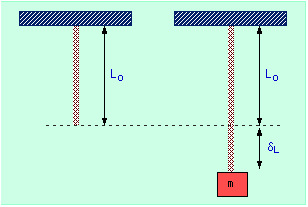# Mass on Vertical Spring homeworkA spring with spring constant k = 40 N/m and unstretched length of L0 is attached to the ceiling. A block of mass m = 2 kg is hung gently on the end of the spring.

a) How far does the spring stretch? dL = 0.49

Now the block is pulled down until the total amount the spring is stretched is twice the amount found in part (a). The block is then pushed upward with an initial speed vi = 2 m/s.
|v|max = ?

F=ma. W=Fd, W = ΔKE, U = mgh, Fs = -Kx

Part a was easy, weight equals spring force so mg = kx => x=mg/k = 0.49.
Part b is where I'm really stuck. Since (U + KE)final = (U + KE)initial then
mgh + 1/2mv^2 = mgh + 1/2mv^2 + Uspring => 2(9.81)(0.981) + 1/2(2)(2^2) = 40(0.981) + 1/2(2)(vmax^2) + 0 so vmax = 4.05 but that's not right. HELP PLEASE!

rl.bhat
Homework Helper
In the second part, total energy in the system with respect to the unstretched position is
mg(2δL) + 1/2*k(2δL)^2 + 1/2mv^2
When it crosses the unstretshed position, it has only KE.
Equating the above two energies find the vmax.

In the second part, total energy in the system with respect to the unstretched position is
mg(2δL) + 1/2*k(2δL)^2 + 1/2mv^2
When it crosses the unstretshed position, it has only KE.
Equating the above two energies find the vmax.

Ok so are you saying that: (mg(2δL) + 1/2*k(2δL)^2 + 1/2mv^2) = 1/2m(vmax)^2, cause when I solve that I get 6.51 which is wrong.

rl.bhat
Homework Helper
Take mg*2δL negative, because it is the work done against the gravity. It will reduce the KE.

Take mg*2δL negative, because it is the work done against the gravity. It will reduce the KE.

Ok so then it should be -(mg(2δL) + 1/2*k(2δL)^2 + 1/2mv^2) = 1/2m(vmax)^2 =>
-2(9.81)(0.98) + .5(40)(.98^2) + .5(2)(2^2) = .5(2)(vmax^2) => vmax = 1.99 but that's wrong too.

rl.bhat
Homework Helper
Now the block is pulled down until the total amount the spring is stretched is twice the amount found in part (a)
So the total stretching from the unstretched position is 3δL.